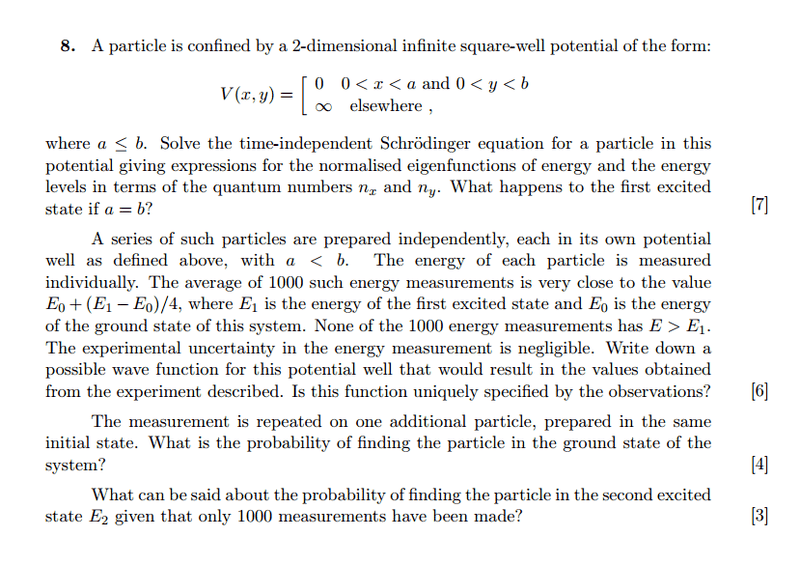# Infinite potential well

unscientific

## Homework StatementPart (a): Find wavefunction and energy levels.
Part (b): Find a possible wavefunction. Is this wavefunction unique?
Part (c): What is the probability of finding it in the ground state?
Part (d): What's the probability of finding it in the second excited state?

## The Attempt at a Solution

Part (a)
I have found the wavefunction and energy levels:

$$\phi = \sqrt{\frac{2}{a}}\sqrt{\frac{2}{a}}sin\left(\frac{n_x \pi x}{a}\right)sin\left(\frac{n_y \pi y}{a}\right)$$

$$E = \frac{\hbar^2}{8ma^2}(n_x^2 + n_y^2)$$

If a = b, there is degeneracy in the first excited state: |nx,ny> |1,0> and |0,1> give the same energy.

Part (b)
$$E = E_0 + \frac{(E_1-E_0)}{4} = \frac{3}{4}E_0 + \frac{1}{4}E_1$$

I'm guessing the wavefunction will be a linear combination of ground and first excited state:

$$|\psi \rangle = \frac{3}{4}|\psi_0\rangle + \frac{1}{4}|\psi_1\rangle$$
$$|\psi \rangle = \sqrt{\frac{2}{a}}\sqrt{\frac{2}{b}}\left[\frac{3}{4} sin(\frac{\pi x}{a})sin(\frac{\pi y}{a}) +\frac{1}{4}sin(\frac{2\pi x}{a})sin(\frac{2\pi y}{a})\right]$$

But the thing is, this wavefunction isn't normalized, as ##(\frac{1}{4})^2 + (\frac{3}{4})^2 = \frac{10}{16}##.

## Answers and Replies

Fisica
i think that $$|\psi \rangle = \frac{3}{4}|\psi_0\rangle + \frac{1}{4}|\psi_1\rangle$$ its wrong

the correct could be $$|\psi \rangle = (\frac{3}{4})^{1/2}|\psi_0\rangle + (\frac{1}{4})^{1/2}|\psi_1\rangle$$

•1 person
skrat
Part (a)
I have found the wavefunction and energy levels:

$$\phi = \sqrt{\frac{2}{a}}\sqrt{\frac{2}{a}}sin\left(\frac{n_x \pi x}{a}\right)sin\left(\frac{n_y \pi y}{a}\right)$$

$$E = \frac{\hbar^2}{8ma^2}(n_x^2 + n_y^2)$$

If a = b, there is degeneracy in the first excited state: |nx,ny> |1,0> and |0,1> give the same energy.

The expression for ##E## seems to be wrong, or is it just me? Is that ##8## in denominator just a typo or... ? You should check that, because I also think ##\pi ## is missing.

Also note that if ##n=0## there is nothing happening. The probability to find a particle is ##|\psi |^2=\frac{2}{a}sin^2(\frac{n\pi }{a}x)=0## if ##n=0## also the energy of state with ##n=0## is zero. Therefore state with ##n=0## does NOT exist. Ground state is ##n=1##.

unscientific
i think that $$|\psi \rangle = \frac{3}{4}|\psi_0\rangle + \frac{1}{4}|\psi_1\rangle$$ its wrong

the correct could be $$|\psi \rangle = (\frac{3}{4})^{1/2}|\psi_0\rangle + (\frac{1}{4})^{1/2}|\psi_1\rangle$$

That's right, because ##E = \langle \psi| H | \psi \rangle##.

But it's not unique, right? Because the state can be exapanded as a series of eigenkets: ##|\psi\rangle = \sum_n a_n |E_n\rangle##.

Last edited:
unscientific
The expression for ##E## seems to be wrong, or is it just me? Is that ##8## in denominator just a typo or... ? You should check that, because I also think ##\pi ## is missing.

Sorry it should be ##h## instead of ##\hbar##. The correct energy is ##E = \frac{h}{8ma^2}##

Also note that if ##n=0## there is nothing happening. The probability to find a particle is ##|\psi |^2=\frac{2}{a}sin^2(\frac{n\pi }{a}x)=0## if ##n=0## also the energy of state with ##n=0## is zero. Therefore state with ##n=0## does NOT exist. Ground state is ##n=1##.

Yea that's right. I think they usually use ##E_0## to denote ground state but the '0' really means n=1 state.

Is the probability ## \frac{3}{4} ## then?

Last edited:
dauto
Also note that the reason the state is not unique is that there is an arbitrary phase ø between the states |ψ> = a|ψ1> + e b|ψ2>.

skrat
Sorry it should be ##h## instead of ##\hbar##. The correct energy is ##E = \frac{h}{8ma^2}##

Close enough. It's actually ##E_n=\frac{\hbar ^2\pi ^2n^2}{2ma^2}=\frac{h^2n^2}{8ma^2}##

Is the probability ## \frac{3}{4} ## then?

Yes, to find/measure the particle with ##E_0## (particle in ground state) the probability is ##\frac{3}{4}##.

unscientific
Close enough. It's actually ##E_n=\frac{\hbar ^2\pi ^2n^2}{2ma^2}=\frac{h^2n^2}{8ma^2}##

Yeah you're right. I keep missing stuff out..

Yes, to find/measure the particle with ##E_0## (particle in ground state) the probability is ##\frac{3}{4}##.

Just that for 4 marks? It seems too easy to be true!

And for part (c), the probability for finding the particle in second excited state is....##\frac{1}{4}##? And again that for 3 marks?!

skrat
Well the problem says that ##E=\frac{3}{4}E_0+\frac{1}{4}E_1## and coefficient before ##E_n## is the probability to find particle in state ##n##.

The problem alsto states that the probability to find a particle in any state with ##n\geq 2## is basically equal to zero. It is trivial to check that, because ##3/4 +1/4 = 1##.

•1 person
dauto
There might be a small probability the state might not be E0 or E1 as long as the probability is small enough that a 1000 particle sample might just have missed it.

•1 person